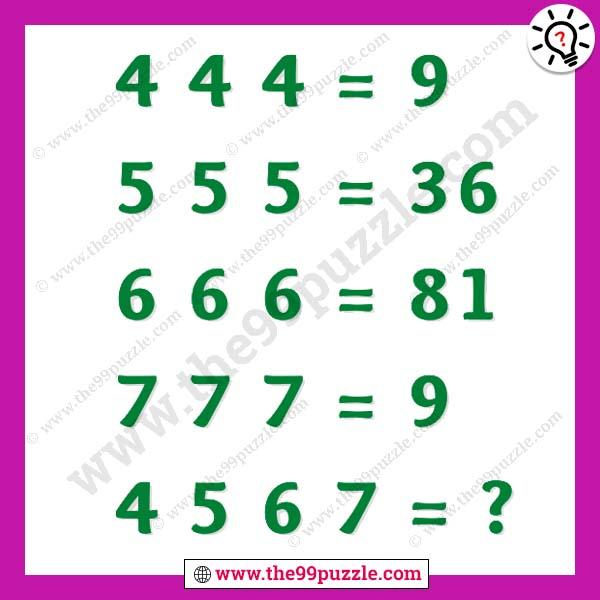# Mind blowing questions for genius with answer – Puzz272

Test your math skill by solving this mathematical puzzle. These are mind-blowing questions for genius people. Every day you can try to solve new puzzles to improve your math skills. The puzzle makes you smarter and more genius. We are sharing many logical math puzzles for all. Learn more math tricks and shortcut math to solve these math puzzles. In this puzzle, you see some numbers in the equation. So, now find out the math logic and replace the question mark.

4 4 4 = 9

5 5 5 = 36

6 6 6 =81

7 7 7 = 9

4 5 6 7 = ?###### Explanation:

4 4 4 = 4+4+4 = 12 = 1+2=3² = 9

5 5 5 = 5+5+5 = 15 = 1+5 = 6² = 36

6 6 6 = 6+6+6 = 36 = 3+6 = 9² = 81

7 7 7 = 7+7+7 = 21 = 2+1 = 3² = 9

4 5 6 7 = 4+5+6+7 = 22 = 2+2 = 4² = 16